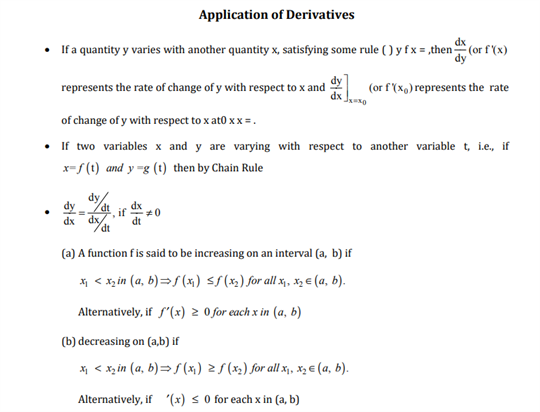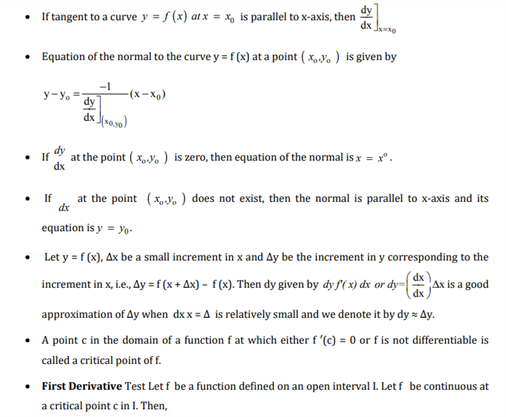# Maths Revision Notes for Class 12 Chapter 6 Application of Derivatives.

The revision notes for chapter 6 is titled as Application of Derivatives. This chapter helps students learn more about solving problems related to the application of derivatives. Some of the major topics that are taught in this chapter are the concepts of derivative as rate of change, equations of tangent and normal, tangent from an external point, angle between the curves, shortest distance between two curves, errors and approximations, definitions etc. Thus, these are some of the various applications of Derivatives which students need to learn, to understand the use of Derivatives in day-to-day life.

Check out the CBSE Class 12 maths revision notes for chapter 6 available below:You may also want to read:

 NCERT Related Articles NCERT Solutions for Class 12 NCERT Solutions for Class 11 NCERT Solutions for Class 10 NCERT Solutions for Class 12 Maths NCERT Solutions for Class 11 Maths NCERT Solutions for Class 10 Maths NCERT Solutions for Class 12 Physics NCERT Solutions for Class 11 Physics NCERT Solutions for Class 10 Science NCERT Solutions for Class 12 Chemistry NCERT Solutions for Class 11 Chemistry NCERT Solutions for Class 6 NCERT Solutions for Class 12 Biology NCERT Solutions for Class 11 Biology NCERT Solutions for Class 6 Maths NCERT Solutions for Class 9 NCERT Solutions for Class 8 NCERT Solutions for Class 6 Science NCERT Solutions for Class 9 Maths NCERT Solutions for Class 8 Maths NCERT Solutions for Class 4 NCERT Solutions for Class 9 Science NCERT Solutions for Class 8 Science NCERT Solutions for Class 4 Maths NCERT Solutions for Class 5 NCERT Solutions for Class 7 NCERT Solutions for Class 7 Maths NCERT Solutions for Class 4 Science NCERT Solutions for Class 5 Maths NCERT Solutions for Class 7 Science NCERT Solutions NCERT Books NCERT Solutions for Class 5 Science